Courses

# Test: Laplace Part - 1

## 10 Questions MCQ Test Signal and System | Test: Laplace Part - 1

Description
This mock test of Test: Laplace Part - 1 for Electrical Engineering (EE) helps you for every Electrical Engineering (EE) entrance exam. This contains 10 Multiple Choice Questions for Electrical Engineering (EE) Test: Laplace Part - 1 (mcq) to study with solutions a complete question bank. The solved questions answers in this Test: Laplace Part - 1 quiz give you a good mix of easy questions and tough questions. Electrical Engineering (EE) students definitely take this Test: Laplace Part - 1 exercise for a better result in the exam. You can find other Test: Laplace Part - 1 extra questions, long questions & short questions for Electrical Engineering (EE) on EduRev as well by searching above.
QUESTION: 1

### Determine the Laplace transform of given signal. Q. x(t) = u( t - 2)

Solution: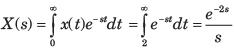QUESTION: 2

### Determine the Laplace transform of given signal. Q. x(t) = u( t + 2)

Solution: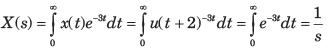QUESTION: 3

### Determine the Laplace transform of given signal. Q. x(t) = e-2tu(t+1)

Solution:

Laplace transform e-at u(t) = 1/s+a

for all Re(s) > - Re(a)

e-2t u(t+1) = 1/(s+2)

QUESTION: 4

Determine the Laplace transform of given signal.
Q. x(t) = e2tu(-t+2)

Solution: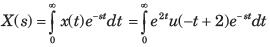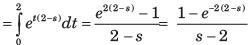QUESTION: 5

Determine the Laplace transform of given signal.
Q. x(t) = sin 5 t

Solution: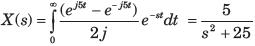QUESTION: 6

Determine the Laplace transform of given signal.
Q. x( t) = u(t) - u(t - 2)

Solution: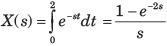QUESTION: 7

Determine the Laplace transform of given signal.
Q. x( t) = d/dt {te-1u(t)}

Solution: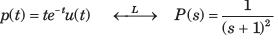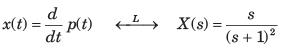QUESTION: 8

Determine the Laplace transform of given signal.
Q. x( t) = tu(t)*cos2πt u(t)

Solution: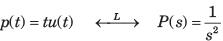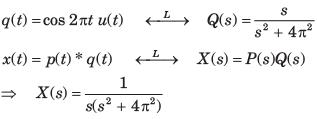QUESTION: 9

Determine the Laplace transform of given signal.
Q. x( t) = t3u(t)

Solution: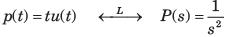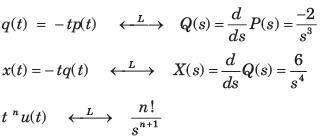QUESTION: 10

Determine the Laplace transform of given signal.
Q. x(t) = u( t - 1) * e-2tu( t - 1)

Solution:

L{−e-at u(t)}

= ∫(∞ to −∞) −e-at u(t)e-stdt

L{−e-at u(t)}

= ∫(∞ to 0) − e-(s+a)t dt

= - |e-(s+a)t/−(s+a)|∞ to 0

= −1/s+a

=> L{−e-2t u(t)} = -1/s+2

=> L{−e-2t u(t-1)} = e-(s+2)/s2 …………(1)

q(t) = L{u(t-1)}

= e-s/(s2)................(2)

Multiply (1) and (2)

= e-2(s+1)/(s+2)